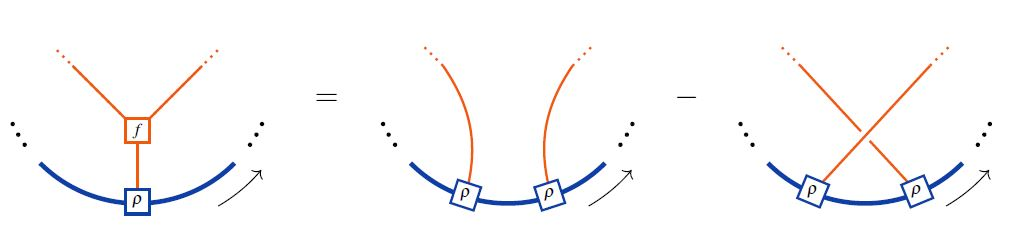# nLab Lie algebra representation

Contents

### Context

#### Representation theory

representation theory

geometric representation theory

## Theorems

#### Lie theory

Background

Smooth structure

Higher groupoids

Lie theory

∞-Lie groupoids

∞-Lie algebroids

Formal Lie groupoids

Cohomology

Homotopy

Related topics

Examples

$\infty$-Lie groupoids

$\infty$-Lie groups

$\infty$-Lie algebroids

$\infty$-Lie algebras

# Contents

## Idea

The representation/action of a Lie algebra $\mathfrak{g}$ on a vector space $V$.

## Definition

A Lie algebra representation is a Lie algebra homomorphism $\rho \;\colon\;\mathfrak{g} \to end(V)$ to the endomorphism Lie algebra of $V$.

This means equivalently that

$\array{ \mathfrak{g} \otimes V & \overset{\rho}{\longrightarrow} & V \\ (x,v) &\mapsto& \rho_x(v) }$

is a bilinear map such that for all $x_i \in \mathfrak{g}$ and $v \in V$ we have the Lie action property:

(1)$\rho_{x_1}(\rho_{x_2}(v)) - \rho_{x_2}(\rho_{x_1}(v)) \;=\; \rho_{[x_1,x_2]}(v)$

## Properties

### In terms of string diagrams / Jacobi diagrams

In string diagram-notation for Lie algebra objects internal to tensor categories, the Lie action property (1) looks as follows:\begin{aligned} \Leftrightarrow & \;\;\;\;\; \rho(f(x,y),z) \;=\; \rho(y,\rho(x,z)) - \rho(x,\rho(y,z)) \\ \underset{ {f = [-,-]} \atop {Lie\;bracket} }{ \Leftrightarrow } & \;\;\;\;\; \underset{ {Lie\;action\;property} }{ \underbrace{ \rho([x,y],z) \;=\; \rho(y,\rho(x,z)) - \rho(x,\rho(y,z)) } } \\ \underset{ {\rho = -[-,-]} \atop {adjoint\;action} }{ \Leftrightarrow } & \;\;\;\;\; \underset{ {Jacobi\;identity} }{ \underbrace{ [[x,y],z] \;=\; - [y,[x,z]] + [x,[y,z]] } } \end{aligned}

Here the last line shows the equivalence to the Jacobi identity on the Lie algebra object itself in the case that the Lie action is the adjoint action.

In the language of Jacobi diagrams this is called the STU-relation, and is the reason behind the existence of Lie algebra weight systems in knot theory.